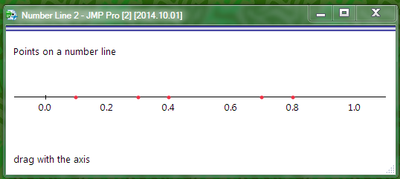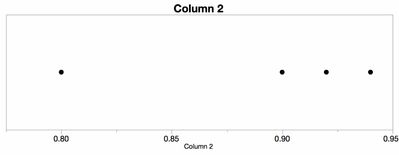It’s World Statistics Day! To honor the theme of the day, the JMP User Community is having conversations about the importance of trust in statistics and data. And we want to hear from you! Tell us the steps you take to ensure that your data is trustworthy.
Choose Language Hide Translation Bar
Highlighted

## Number line

How do I create a simple elementary school type number line in JMP?  I'd like to plot numbers such as 0.8, 0.9, 0.92, 0.94 on a number line.  I've created a chart, using data statistics with the horizontal & point chart options--how do I get rid of the Y axis so the values will be plotted on the X axis only?

1 ACCEPTED SOLUTION

Accepted Solutions
Highlighted

## Re: Number line

same idea, using JSL.  Looks sharp if the graph background matches the window background.

Making Anonymous Graphs in JMP 8 - JMP Blog has some secrets for removing extra lines and text from a graph, reused here.

JMP 11 lets the vertical axis be very short, which helps the appearance.  Two reference lines, both at zero, look like axes.`dt = New Table( "Untitled", New Column( "x", Set Values( [0.1, 0.8, 0.7, 0.3, 0.4] ) ) );xvals = dt:x << get values;yvals = J( N Rows( xvals ), 1, 0 );New Window( "Number Line",    BorderBox(left(10),top(10),right(10),bottom(10), vlistbox(    Text Box( "Points on a number line" ),    Spacer Box( size( 10, 50 ) ),    g = Graph Box( Frame Size( 500, 7 ),        X Scale( -0.1, 1.1 ), Y Scale( -1, 1 ),        yname( " " ), Marker Size( 2 ),         yaxis( show major ticks( false ), show minor ticks( false ),            show labels( false ), Inside Ticks( 1 ),            Add Ref Line( 0.0, Solid, "Black", "", 1 ) ),        xaxis( show major ticks( false ), show minor ticks( false ),            show labels( true ), Add Ref Line( 0.0, Solid, "Black", "", 1 ),            Inside Ticks( 1 ) ),        Marker( Combine States( Marker State( 12 ),color state("red") ), xvals, yvals )    ),    Spacer Box( size( 10, 50 ) ),    Text Box( "drag with the axis" )    )));// http://blogs.sas.com/content/jmp/2008/11/10/making-anonymous-graphs-in-jmp-8/g[axis box( 1 )] << remove axis label;g[axis box( 2 )] << remove axis label;// hide the inner borderg[frame box( 1 )] << Left( 0 ); // no line on left, etcg[frame box( 1 )] << Right( 0 );g[frame box( 1 )] << top( 0 );g[frame box( 1 )] << bottom( 0 );// shrink and hide the outer border toog[Border Box( 1 )] << Left( 0 ); // no space on left, etcg[Border Box( 1 )] << Right( 0 );g[Border Box( 1 )] << Top( 0 );g[Border Box( 1 )] << bottom( 0 );g[Border Box( 1 )] << sides( 0 ); // draw no sides (1+2+4+8 would be all four sides)`
Craige
2 REPLIES 2

## Re: Number line

Try graph builder, just drag your column to the x-axis.Highlighted

## Re: Number line

same idea, using JSL.  Looks sharp if the graph background matches the window background.

Making Anonymous Graphs in JMP 8 - JMP Blog has some secrets for removing extra lines and text from a graph, reused here.

JMP 11 lets the vertical axis be very short, which helps the appearance.  Two reference lines, both at zero, look like axes.`dt = New Table( "Untitled", New Column( "x", Set Values( [0.1, 0.8, 0.7, 0.3, 0.4] ) ) );xvals = dt:x << get values;yvals = J( N Rows( xvals ), 1, 0 );New Window( "Number Line",    BorderBox(left(10),top(10),right(10),bottom(10), vlistbox(    Text Box( "Points on a number line" ),    Spacer Box( size( 10, 50 ) ),    g = Graph Box( Frame Size( 500, 7 ),        X Scale( -0.1, 1.1 ), Y Scale( -1, 1 ),        yname( " " ), Marker Size( 2 ),         yaxis( show major ticks( false ), show minor ticks( false ),            show labels( false ), Inside Ticks( 1 ),            Add Ref Line( 0.0, Solid, "Black", "", 1 ) ),        xaxis( show major ticks( false ), show minor ticks( false ),            show labels( true ), Add Ref Line( 0.0, Solid, "Black", "", 1 ),            Inside Ticks( 1 ) ),        Marker( Combine States( Marker State( 12 ),color state("red") ), xvals, yvals )    ),    Spacer Box( size( 10, 50 ) ),    Text Box( "drag with the axis" )    )));// http://blogs.sas.com/content/jmp/2008/11/10/making-anonymous-graphs-in-jmp-8/g[axis box( 1 )] << remove axis label;g[axis box( 2 )] << remove axis label;// hide the inner borderg[frame box( 1 )] << Left( 0 ); // no line on left, etcg[frame box( 1 )] << Right( 0 );g[frame box( 1 )] << top( 0 );g[frame box( 1 )] << bottom( 0 );// shrink and hide the outer border toog[Border Box( 1 )] << Left( 0 ); // no space on left, etcg[Border Box( 1 )] << Right( 0 );g[Border Box( 1 )] << Top( 0 );g[Border Box( 1 )] << bottom( 0 );g[Border Box( 1 )] << sides( 0 ); // draw no sides (1+2+4+8 would be all four sides)`
Craige
Article Labels

There are no labels assigned to this post.